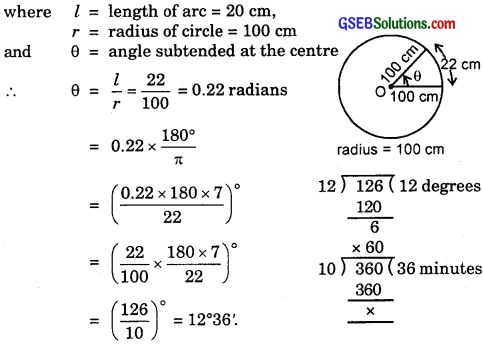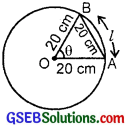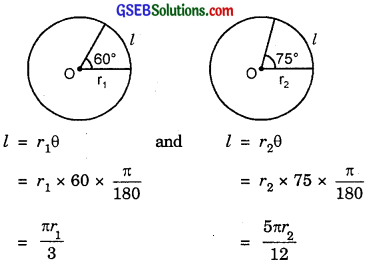# GSEB Solutions Class 11 Maths Chapter 3 Trigonometric Functions Ex 3.1

Gujarat Board GSEB Textbook Solutions Class 11 Maths Chapter 3 Trigonometric Functions Ex 3.1 Textbook Questions and Answers.

## Gujarat Board Textbook Solutions Class 11 Maths Chapter 3 Trigonometric Functions Ex 3.1

Question 1.
Find the radian measures corresponding to the following degree measures?

1. 25°
2. – 47°30′
3. 240°
4. 520°

Solution:
1. We have:
∴ 25° = $$\frac{π}{180}$$ × 25 radians = $$\frac{5π}{36}$$ radians.

2. 60′ = 1°
∴ 30′ = $$\frac{30°}{60}$$ = $$\frac{1°}{2}$$
∴ 47°30′ = (47 + $$\frac{1}{2}$$) degrees = $$\frac{95}{2}$$ degrees
So, – $$\frac{95°}{2}$$ = $$\frac{- π}{180}$$ × $$\frac{95}{2}$$ radians = $$\frac{- 19π}{72}$$ radians.

∴ 240° = $$\frac{π}{180}$$ × 240 radians
= $$\frac{4π}{3}$$ radians.

∴ 520° = $$\frac{π}{180}$$ × 520 radians = $$\frac{26π}{9}$$ radians.Question 2.
Find the degree measures corresponding to the following radian measures. [Use π = $$\frac{22}{7}$$]
(i) $$\frac{11}{16}$$
(ii) – 4
(iii) $$\frac{5π}{3}$$
(iv) $$\frac{7π}{6}$$
Solution:
(i) π radians = $$\frac{22}{7}$$ radians = 180°
∴ $$\frac{11}{16}$$ radians = $$\frac{180}{22}$$ × 7 × $$\frac{11}{16}$$ degree
= $$\frac{315}{8}$$ degrees = 39 $$\frac{3}{8}$$ degrees
= 39°22’30”.
Note: $$\frac{3°}{8}$$ = $$\frac{3}{8}$$ × 60′ = $$\frac{45}{2}$$ = 22’30”.

(ii)(iii) $$\frac{5π}{3}$$ = $$\frac{5}{3}$$ × 180° = 300°.

(iv) $$\frac{7π}{6}$$ = $$\frac{7}{6}$$ × 180° = 210°.Question 3.
A wheel makes 360 revolutions in one minute. Through how many radians does it turn in one second?
Solution:
Angle rotated in one revolution = 2π radians
∴ Angle rotated in 360 revolutions = 360 × 2π radians
⇒ Angle turned in one minute or 60 sec = 360 × 2π.
Hence, angle turned in 1 sec = $$\frac{360 × 2π}{60}$$.

Question 4.
Find the degree measure of the angle subtended at the centre of a circle of radius 100 cm by an arc of length 22 cm. (Use π = $$\frac{22}{7}$$)
Solution:
We know that:
l = rθ,
where l = length of arc = 20 cm,
r = radius of circle = 100 cm
and θ = angle subtended at the centreQuestion 5.
In a circle of diameter 40 cm, the length of a chord is 20 cm. Find the length of the minor arc corresponding to the chord?
Solution:
Since radius = length of chord = 20 cm, so
∆ OAB is equilateral triangle
⇒ θ = 60°.
Now, l = rθ
So, l = 20 × 60° × $$\frac{π}{180°}$$ = $$\frac{20π}{3}$$
Thus, l = $$\frac{20π}{3}$$ cm.Question 6.
If, in two circles, arcs of the same length subtend angles of 60° and 75° at their centres, find the ratio of their radii?
Solution:Since l is same for both the circles, therefore
$$\frac{π}{3}$$r1 = $$\frac{5π}{12}$$r2.
⇒ r1 : r2 = 5 : 4.Question 7.
Find the angle in radians through which a pendulum swings, if its length is 75 cm and the tip describes an arc of length:

1. 10 cm
2. 15 cm
3. 21 cm

Solution:
1. r = 75 cm,
l = 10 cm,
θ = ?
⇒ θ = $$\frac{l}{r}$$ = $$\frac{10}{75}$$ radians.
= $$\frac{2}{15}$$ radians

2. r = 75 cm,
l = 15 cm,
∴ θ = $$\frac{l}{r}$$ = $$\frac{15}{75}$$ radians = $$\frac{1}{5}$$ radians.

3. r = 75 cm,
l = 21 cm,
∴ θ = $$\frac{l}{r}$$ = $$\frac{21}{75}$$ radians = $$\frac{7}{25}$$ radians.